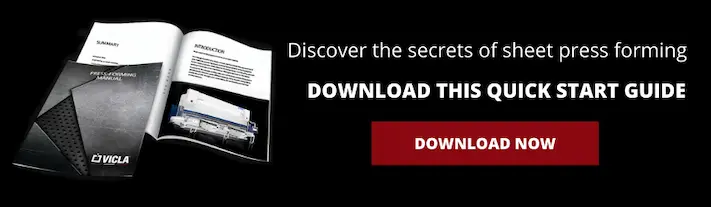# SHEET METAL K-FACTOR: WHAT IT IS AND HOW TO CALCULATE ITThe k-factor is fundamental in the press-bending sector and is closely linked to the concept of spring back. It is also known as bend allowance and serves to calculate the sheet metal layout. Knowing the k-factor formula is essential in order to bend any kind of sheet metal correctly; in fact, it varies according to the type of material to be deformed.

## What is the k-factor?

The k-factor indicates the behaviour of the material being bent and how it reacts to the applied tensile and compressive stresses.
In essence, it's nothing more than the ratio of the sheet metal thickness to the neutral axis, i.e. between t (distance between the inside of the bend and the neutral plane) and T (the workpiece thickness).

## What is the neutral plane?

The neutral plane is the ideal area that does not vary during bending, and neither shortens nor lengthens. Do you want to know more about the neutral plane? We have written an article that goes into this topic in more detail.
Broadly speaking, we can say that in the vast majority of cases the neutral plane lies at about 1/3 of the thickness from the inside, so it is 0.33.

E.g.: a thickness of 30/10 will have t = 1 mm, so 1/3 = 0.33 = k
Nevertheless, there are cases in which the neutral radius tends to shift towards the centre, i.e. when the ratio

r/T > 1

Where r is the inside bend radius and T is the thickness.

## K-factor formula for sheet metal

The following formula is used to calculate the sheet metal layout:

k = log(r/s)x0.5+0.65

In any case, this table provides a set of values that can be used once you have the right inside bend radius.

### Sheet metal k-factor table

 0,65>r/t<=1 k=0.3 1 k=0.35 1.5 k=0.4 2.4 k=0.45 3.8 k=0.5

What affects the sheet metal layout?

The sheet metal layout generally depends on factors such as: the material, thickness and dies used and, to a lesser extent, the punch radius.
All of these components affect the inside radius to varying extents. The natural consequence is that, the larger the radius, the smaller the layout, and vice versa.

### Let’s now look at the main factors that affect the sheet metal layout:

1) The die width: a wider die creates a larger radius than a narrower one.
2) Bend type: another aspect that is often ignored is that the finished workpiece dimensions vary for the same die, material and thickness, depending on whether air bending or bottom bending is used. In fact, the latter creates a smaller radius and therefore requires longer layouts.
Special consideration must be given to coining. It is the only method in which the inside radius is the same as the punch radius, so the layout depends on the punch radius alone.
3) Punch radius: as already seen, the punch radius in standard conditions (therefore not with special bends that require the use of tools with very large radii) should be about 2/3 of the inside bend radius.

This is because, to a lesser extent, this component also tends to create differences in the dimensions of finished workpieces.

Bending medium thicknesses with a punch radius that is too small (e.g. r of 0.8 on a thickness of 50/10) not only creates unsightly grooves on the inside, but also causes more material stress and an unnatural curvature.

## Sheet metal k-factor: how to lay out a metal sheet

Workshops use various techniques to find the layout.
There is now a tendency to rely on the many software packages on the market, but the corrections made may still be based on empirical methods developed within companies.
Now let us look at some of the methods used by operators.

• Experience-based tables: these are obtained by noting the compensation added to layouts in practical trials.
• Compensation values: this involves adding compensation values corresponding to a percentage of the thickness to the inside bend measurements.
• DIN tables: these are tables that provide values to be subtracted from the outside dimensions of the bent workpieces.
• Geometric calculations: by applying the k-factors obtained from the formula seen above or by using the example grid values based on r/t.
• Forcing the radius and changing the k-factor: this is an approach used by designers and draughtsmen who use 3D modelling software.
• Percentage method: this is a widely used system. It involves initially simplifying the inside radius of the alloy based on the die and material, and then entering the k-factor from the table.

Now that you know what the k-factor is in detail and how to calculate it, you can accurately determine the layout of the sheet metal to be bent.